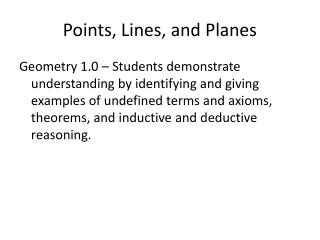DownloadDownload PresentationPoints, Lines, and Planes

# Points, Lines, and Planes

Télécharger la présentation## Points, Lines, and Planes

- - - - - - - - - - - - - - - - - - - - - - - - - - - E N D - - - - - - - - - - - - - - - - - - - - - - - - - - -
##### Presentation Transcript

1. Points, Lines, and Planes Geometry 1.0 – Students demonstrate understanding by identifying and giving examples of undefined terms and axioms, theorems, and inductive and deductive reasoning.

2. Points, Lines, & Planes No Bell-Work today, just take out your notebook and pencil, have your letter to parent out for me to collect. You will organize your notebook. 3. We will begin our first lesson.

3. Postulate 1-1 Through any two points there is exactly one line. Line t is the only line that passes through points A and B. Points, Lines, and Planes

4. Postulate 1-2 If two lines intersect, then they intersect in exactly one point. AE and BD intersect at C.

5. Collinear points are points that lie on the same line. • Are the three points collinear? • A, D, E 5. C, B, D • A, B, F 6. A, E, C • F, B, D 7. F, E, A • G, F, C 8. A, G, C A B D C E G F

6. Bell Work 2- x 4 – VW and plane VWY

7. Plane is a flat surface that has no thickness. Coplanar are points and lines on the same plane.

8. Postulate 1-3 If two planes intersect, then they intersect in exactly one line. Plane RST and plane STW intersect in ST.

9. Name the intersection of each pair of plane. C B • Plane ABC and RSD • Plane UXS and DCS • Plane ABU and RUX • Plane SXC and BCU D A X U S R

10. Write coplanar or non-coplanar • A, B, C, S • X, Y, Z, U • S, U, V, Y • X, S, V, U